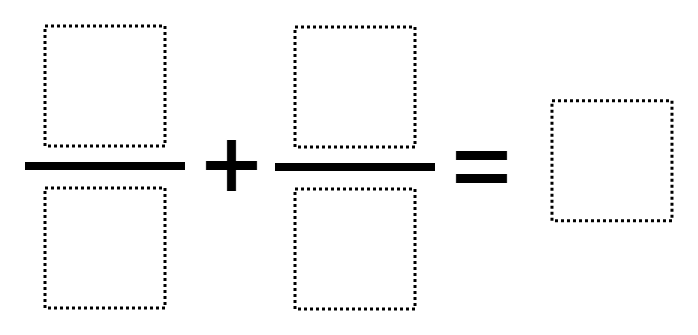Home > Grade 5 > Adding Fractions to Make a Whole Number

# Adding Fractions to Make a Whole Number

Directions: Using the digits 1-9, fill in the blanks to make a whole number sum. Use each digit only at most one time. Can you make all whole numbers from 1 to 9?### Hint

Are you using equivalent fractions?

Number of Unique Solutions: 36
1: 2 / 1 + 6 / 3 = 4
2: 2 / 1 + 9 / 3 = 5
3: 3 / 1 + 8 / 4 = 5
4: 3 / 4 + 2 / 8 = 1
5: 3 / 6 + 2 / 4 = 1
6: 3 / 6 + 7 / 2 = 4
7: 3 / 6 + 9 / 2 = 5
8: 4 / 2 + 3 / 1 = 5
9: 4 / 2 + 5 / 1 = 7
10: 4 / 2 + 6 / 1 = 8
11: 4 / 2 + 7 / 1 = 9
12: 4 / 6 + 3 / 9 = 1
13: 4 / 8 + 3 / 6 = 1
14: 5 / 1 + 6 / 3 = 7
15: 5 / 1 + 8 / 2 = 9
16: 5 / 1 + 8 / 4 = 7
17: 5 / 2 + 4 / 8 = 3
18: 5 / 2 + 9 / 6 = 4
19: 6 / 2 + 4 / 1 = 7
20: 6 / 2 + 5 / 1 = 8
21: 6 / 4 + 7 / 2 = 5
22: 6 / 8 + 5 / 4 = 2
23: 6 / 9 + 4 / 3 = 2
24: 7 / 1 + 6 / 3 = 9
25: 7 / 2 + 9 / 6 = 5
26: 8 / 2 + 3 / 1 = 7
27: 8 / 2 + 9 / 3 = 7
28: 8 / 4 + 6 / 2 = 5
29: 8 / 4 + 7 / 1 = 9
30: 9 / 2 + 4 / 8 = 5
31: 9 / 3 + 4 / 1 = 7
32: 9 / 3 + 4 / 2 = 5
33: 9 / 3 + 5 / 1 = 8
34: 9 / 3 + 8 / 4 = 5
35: 9 / 4 + 6 / 8 = 3
36: 9 / 6 + 4 / 8 = 2

Source: Owen Kaplinsky

## Balanced Equations 2

Directions: Use the operation symbols (+, -, x, ÷) and equal sign (=) to make …

1.Again, no fill in the blanks picture is shown.

•Sorry. Just fixed it.

2.I have not been able to find the 1-9 digit cards for the activities. Where can I find them?

•We don’t currently have anything like that. You could have your kids make their own.

•We use color tiles with the digits written on them in permanent marker. They work great! If you need an image let me know.

•Cindy, we just added them to the site on the top right side under “Number Tiles”

•Or, have students put this paper/handout into a game sleeve and have them do trial and error using a dry and erase marker.

3.kzjhdbdouysegfcjhdbliywecjeiynsdbcvcnsdgdsjd

4.There’s no six in the answer key

5.3/4+2/8=1

6.With 9 possible digits to choose from and 5 places to put them, there are 9P5 = 15,120 unique permutations for ways to arrange the numbers. Most of those permutations obviously result in non-integer solutions.

7.3/6 + 2/4 = 1

8.